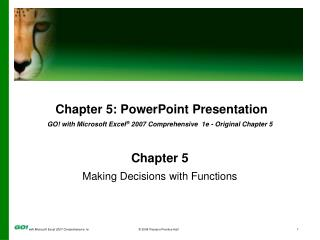DownloadDownload PresentationChapter 5 : PowerPoint Presentation

# Chapter 5 : PowerPoint Presentation

Download Presentation## Chapter 5 : PowerPoint Presentation

- - - - - - - - - - - - - - - - - - - - - - - - - - - E N D - - - - - - - - - - - - - - - - - - - - - - - - - - -
##### Presentation Transcript

1. Chapter 5: PowerPoint Presentation GO! with Microsoft Excel® 2007 Comprehensive 1e - Original Chapter 5 Chapter 5 Making Decisions with Functions

2. Objectives • Create Text Functions • Create Statistical Functions • Insert Date and Time Functions • Create Logical Functions and Insert a Comment

3. Objectives • Insert Financial Functions • Create What-If Analysis with Goal Seek • Determine Future Value and Present Value

4. Create Text Functions • Functions are built-in formulas that complete tasks quickly. • Functions are usually used for complex mathematical calculations. • They are also used for formatting purposes.

5. Create Text Functions • Text functions, such as the TRIM and PROPER functions, format text or add text to a cell. • The PROPER function capitalizes the first letter of each word in a cell entry. • The TRIM functionremoves all spaces from text except for single spaces between words.

6. Create Text Functions • =UPPER(A2) Changes text to all UPPERCASE (NANCY DAVOLIO) • =LOWER(A2) Changes text to all lowercase (nancy davolio) • =PROPER(A2) Changes text to Title Case (Nancy Davolio)

7. Create Statistical Functions • Statistical functions provide statistical analysis of a worksheet. • The median is the value at the midpoint of a series of numbers. • The mode is the value that occurs the most often in a list of numbers.

8. Create Statistical Functions • After you type an = (equal sign) and the beginning letters of a function, Excel displays a dynamic list of valid functions. List of function choices

9. Create Statistical Functions • The COUNT function counts the number of cells in the selected range that contain numeric values. • The COUNTA function counts the number of cells in the range that are not empty.

10. Insert Date and Time Functions • Date and time functions can be static or volatile. • A static date or time remains the same and does not change. • A volatile date or time is updated each time the worksheet is used.

11. Insert Date and Time Functions • The TODAY function returns the current date. Each time the worksheet is opened, the TODAY function recalculates so the current date displays. • Click the Formulas tab, and in the Function Library group, click the Date and Time button. From the displayed list click TODAY.

12. Create Logical Functions and Insert a Comment • Logical functions test a logical comparison between expressions. • They determine whether the conditions are true or false.

13. Create Logical Functions and Insert a Comment • The AND function tests two or more arguments to see whether they are true or false. • Returns TRUE if all arguments are true. • Returns FALSE if any argument is not true. • The OR function tests two or more arguments to see whether they are true or false and returns TRUE when any one of the arguments is true.

14. Create Logical Functions and Insert a Comment • Comments can provide additional information that explains the data in a worksheet. • A comment is a remark that is attached to a specific cell but does not display with the worksheet. • An indicator alerts the user that a comment is attached to the cell.

15. Create Logical Functions and Insert a Comment • Printing comments: • Comments can be printed as they are displayed. • Or they can be printed as text at the end of the worksheet.

16. Create Logical Functions and Insert a Comment Comment Comments group Red triangle indicates comment is in cell

17. Insert Financial Functions • Excel provides more than 50 financial functions. • Three functions that are frequently used are: • Future value: FV (rate, periods, payment) • Payment: PMT (rate, periods, loan amount) • Present value: PV (rate, periods, payment)

18. Insert Financial Functions Formulas tab Required arguments Name of function

19. Create What-If Analysis with Goal Seek • Goal Seek is one of Excel’s “what-if” analysis tools. • It’s used to find a specific value for a cell by adjusting the value in another cell.

20. Create What-If Analysis with Goal Seek • To use Goal Seek: • Select the Data tab. • In the Data Tools group, click the What-If Analysis button. • In the displayed list, click Goal Seek.

21. Create What-If Analysis with Goal Seek What-If Analysis button Data Tools group Goal Seek dialog box

22. Determine Future Value and Present Value • Two financial functions that are used frequently in business are FV and PV: • Future value (FV)is the amount that an investment made today will be worth in the future. • Present value (PV) is the amount of money invested now in order to have a specific amount of money at a future time.

23. Covered Objectives • Create Text Functions • Create Statistical Functions • Insert Date and Time Functions • Create Logical Functions and Insert a Comment

24. Covered Objectives • Insert Financial Functions • Create What-If Analysis with Goal Seek • Determine Future Value and Present Value